ISEE Middle Level Math : How to find the perimeter of square

Example Questions

← Previous 1 3

Example Question #1301 : Concepts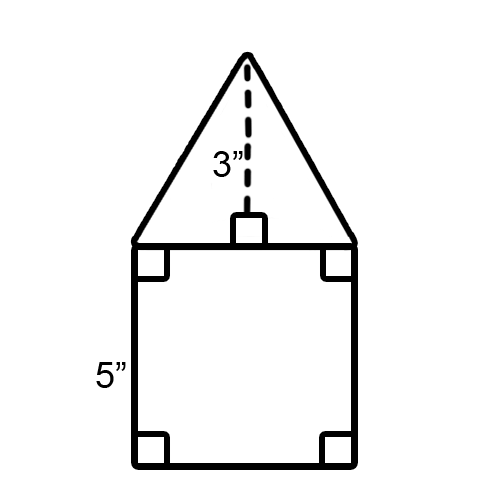Use this image for the following problem.

What is the perimeter of the square in this picture?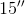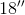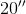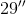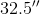Explanation:

The question only is looking for a part of the picture, just the square. With squares, the rule is that all the sides are equivalent, meaning the same lengths and all angles are right angles.

Perimeter means adding up all the sides together. So we just need to add the lengths of the sides of the square. Uh oh, we only have one side that is listed.

Again, remember that with squares the sides are equivalent, and we know one side is 5 inches. We just need to take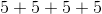because a square has 4 sides.

Our perimeter is.

Example Question #1 : How To Find The Perimeter Of Square

Sandy wants to put a border around her son’s nursery. If all four square walls in the room have the same width and use upfeet of border, what is the length of one wall?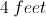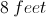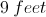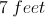Explanation:

When Sandy puts the border around her son's room, she will need enough to cover the perimeter.  Since the room has four walls equal in length, we know that the room is a square.  The perimeter of a square can by found by adding all the sides together, or by multiplying the length of one side by 4.  This can be written as: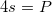Since we know that Sandy usedfeet of border, we know the perimeter is. We can now write an equation: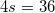Now, in order to isolate the variable, we can divide both sides by four.

The left-hand side simplifies to: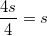The right-hand side simplifies to: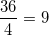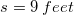When we solve, we find that the length of each wall is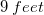.

Example Question #3 : Squares

Find the perimenter: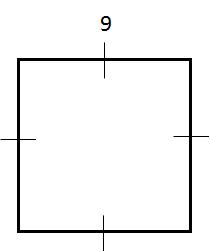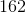Explanation:

The perimeter is equal to the sum of the length of all sides. Each side is equal to. Therefore, the perimeter equals: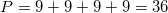Example Question #1 : How To Find The Perimeter Of Square

Find the perimeter of a square with side length 10.Explanation:

To solve, simply multiply the side length by 4 since all sides in a square area equal. Thus,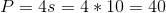Example Question #5 : Squares

A new building in New York City will have a footprint that is a perfect square, taking up a complete city block. If one city block is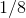of a mile, what is the perimeter of the building?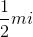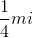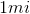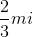Explanation:

A new building in New York City will have a footprint that is a perfect square, taking up a complete city block. If one city block isof a mile, what is the perimeter of the building?

To find the perimeter of a square, we need to multiply the side length by 4.

Our side length ismile, so do the following to find our answer: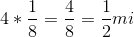So the perimeter isExample Question #6 : Squares

Use the following to answer the question.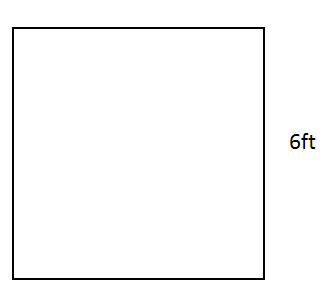Find the perimeter of the square.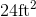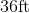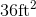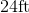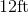Explanation:

To find the perimeter of a square, we use the following formula: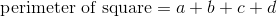where a, b, c, and d are the lengths of each side.

Now, we know that a square has equal sides.  So given the squarewe know that each side equals 6ft.  So, we can use this to substitute into the formula.  We get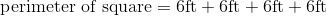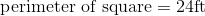Example Question #7 : Squares

Find the perimeter of a square with a width of 8 inches.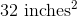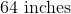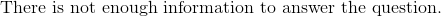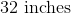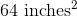Explanation:

To find the perimeter of a square, we use the following formula:where a, b, c, and d are the lengths of the sides of the square.

Now, we know the width of the square is 8 inches.  Because it is a square, we know all sides are equal.  Therefore, all sides are 8 inches.  Knowing this, we can substitute into the formula.  We get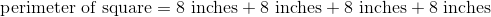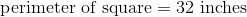Example Question #8 : Squares

You are playing Monopoly and notice that the dice is a cube. If the dice has a height of 2 centimeters, what is the perimeter of one of its surfaces?

Cannot be determined from the information provided.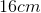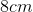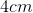Explanation:

You are playing Monopoly and notice that the dice is a cube. If the dice has a height of 2 centimeters, what is the perimeter of one of its surfaces?

Begin by realizing that we need to find the perimeter of a square.

Next, recall that the height of a cube is the same as its length and width. In other words, one surface of a cube will be a square with sides equal to the height of the cube.

Armed with this knowledge, we can find the perimeter of the square.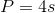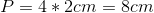So our answer is 8cm

Example Question #9 : Squares

A square stamp has an edge length of 6 centimeters, what is its perimeter?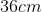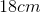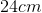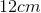Explanation:

A square stamp has an edge length of 6 centimeters, what is its perimeter?

To find the perimeter of a square, we simply can multiply the edge length by 4.

Doing so yields: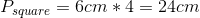Example Question #1 : Quadrilaterals

Find the perimeter of a square that has a base of length 9 inches.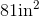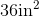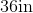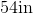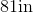Explanation:

To find the perimeter of a square, we will use the following formula:where a, b, c, and d are the lengths of each side of the square.

Now, we know the base has a length of 9 inches.  Because it is a square, we know that all sides are equal.  Therefore, all sides are 9 inches.  Knowing this, we can substitute into the formula.  We get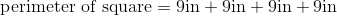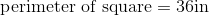← Previous 1 3

All ISEE Middle Level Math Resources